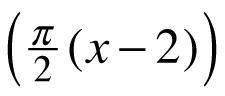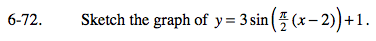### Home > PC > Chapter 6 > Lesson 6.2.1 > Problem6-72

6-72.

Sketch the graph of y = 3 sin+ 1. Homework Help ✎The amplitude is 3 and the vertical shift is up 1, therefore the range of the function is −2 ≤ y ≤ 4.
Sketch maximum and minimum lines at y = −2 and y = 4 to help you bound the curve.
Also, sketch the midline at y = 1.

The curve is shifted right 2 units.
Since this is a sine function, it starts in the middle and goes up.
Put a locator point on the midline at x = 2.

Since b = π/2, the period is 2π/b or 4.
Put another point on the midline 4 units to the right (or left) of the first point you marked.

Plot the remaining three points needed to sketch the curve.
One should be in the middle of your first two and on the midline.
One should be a maximum in the middle of the first and middle points.
The other should be a minimum in the middle of the middle and last points.